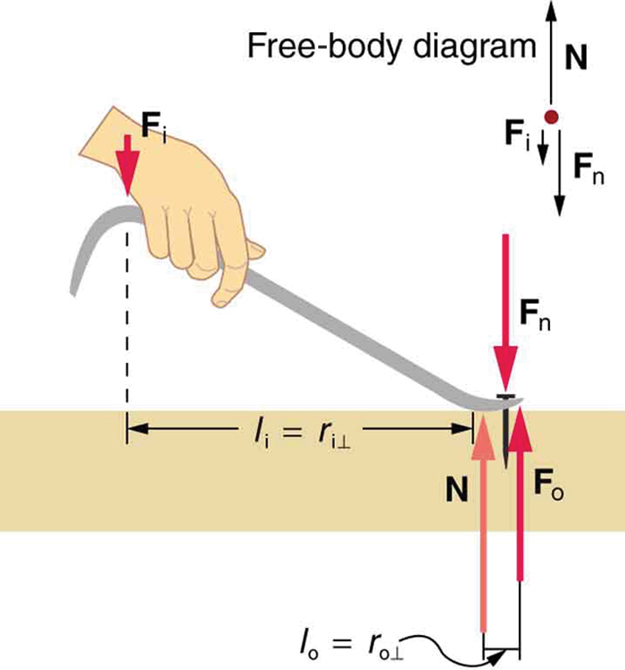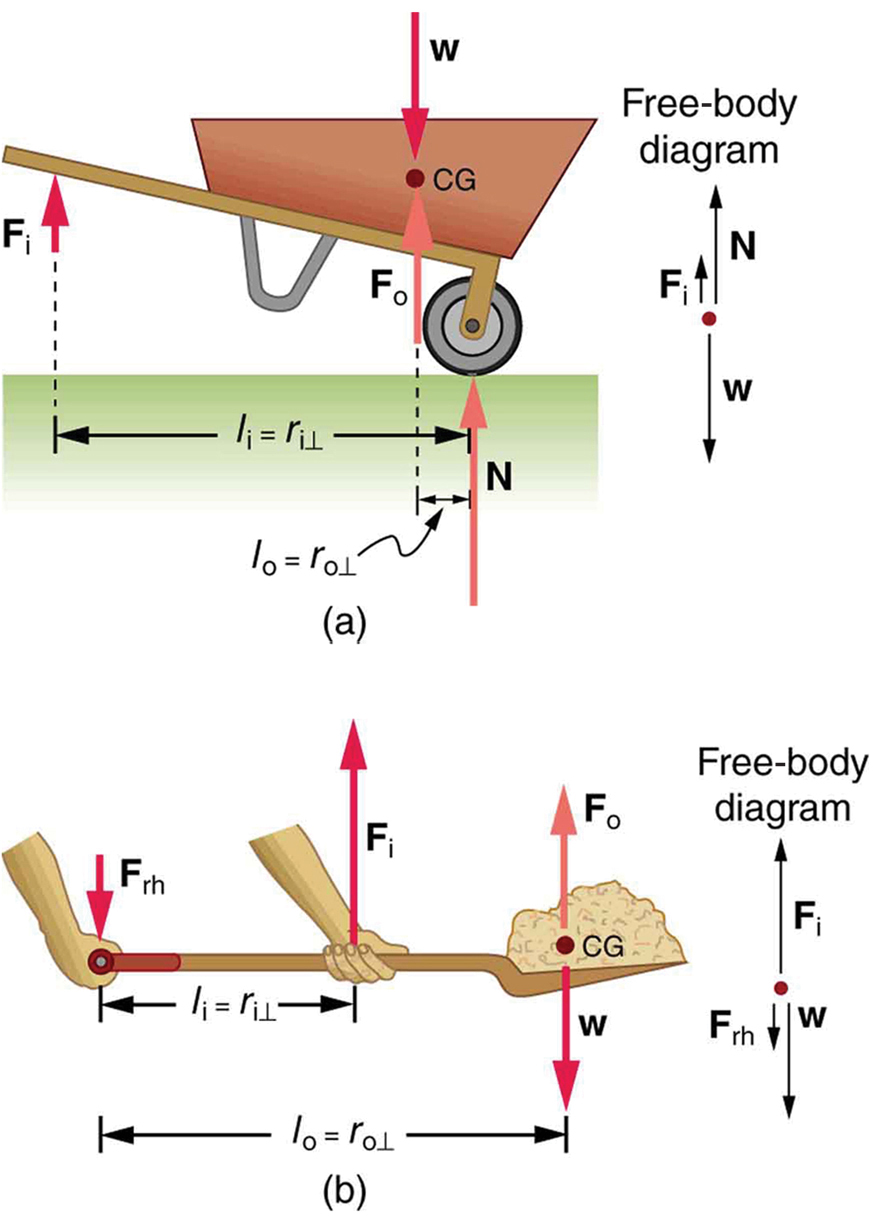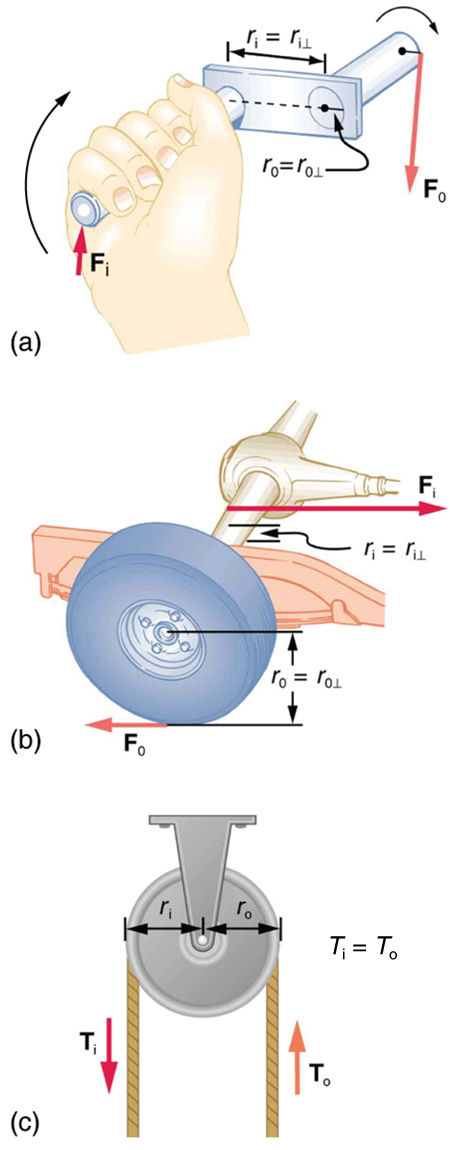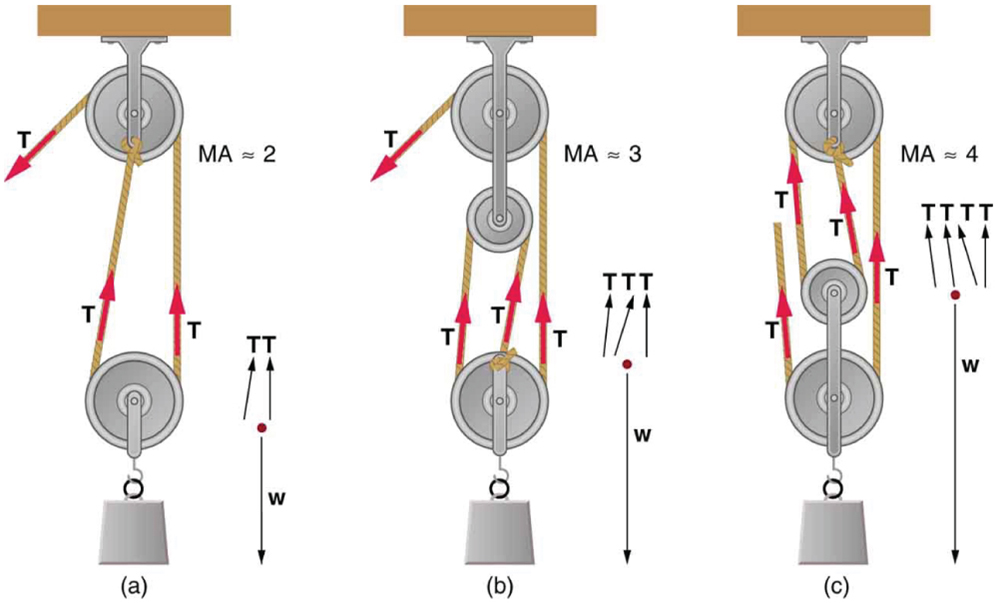Chapter 9 Statics and Torque

# 9.5 Simple Machines

### Summary

• Describe different simple machines.

Simple machines are devices that can be used to multiply or augment a force that we apply – often at the expense of a distance through which we apply the force. The word for “machine” comes from the Greek word meaning “to help make things easier.” Levers, gears, pulleys, wedges, and screws are some examples of machines. Energy is still conserved for these devices because a machine cannot do more work than the energy put into it. However, machines can reduce the input force that is needed to perform the job. The ratio of output to input force magnitudes for any simple machine is called its mechanical advantage (MA).

$\boldsymbol{\textbf{MA}\:=}$$\boldsymbol{\frac{F_{\textbf{o}}}{F_{\textbf{i}}}}$

One of the simplest machines is the lever, which is a rigid bar pivoted at a fixed place called the fulcrum. Torques are involved in levers, since there is rotation about a pivot point. Distances from the physical pivot of the lever are crucial, and we can obtain a useful expression for the MA in terms of these distances.Figure 1. A nail puller is a lever with a large mechanical advantage. The external forces on the nail puller are represented by solid arrows. The force that the nail puller applies to the nail (Fo) is not a force on the nail puller. The reaction force the nail exerts back on the puller (Fn) is an external force and is equal and opposite to Fo. The perpendicular lever arms of the input and output forces are li and l0.

Figure 1 shows a lever type that is used as a nail puller. Crowbars, seesaws, and other such levers are all analogous to this one.$\boldsymbol{\textbf{F}_{\textbf{i}}}$is the input force and$\boldsymbol{\textbf{F}_{\textbf{o}}}$is the output force. There are three vertical forces acting on the nail puller (the system of interest) – these are$\boldsymbol{\textbf{F}_{\textbf{i}},\: \textbf{F}_{\textbf{o}}}$and$\textbf{N}.$$\boldsymbol{\textbf{F}_{\textbf{n}}}$is the reaction force back on the system, equal and opposite to$\boldsymbol{\textbf{F}_{\textbf{o}}}.$(Note that$\boldsymbol{\textbf{F}_{\textbf{o}}}$is not a force on the system.)$\textbf{N}$is the normal force upon the lever, and its torque is zero since it is exerted at the pivot. The torques due to$\boldsymbol{\textbf{F}_{\textbf{i}}}$and$\boldsymbol{\textbf{F}_{\textbf{n}}}$must be equal to each other if the nail is not moving, to satisfy the second condition for equilibrium$\boldsymbol{(\textbf{ net }\tau=0)}.$(In order for the nail to actually move, the torque due to$\boldsymbol{\textbf{F}_{\textbf{i}}}$must be ever-so-slightly greater than torque due to$\boldsymbol{\textbf{F}_{\textbf{n}}}$.) Hence,

$\boldsymbol{l_{\textbf{i}}F_{\textbf{i}}=l_{\textbf{o}}F_{\textbf{o}}}$

where$\boldsymbol{l_{\textbf{i}}}$and$\boldsymbol{l_{\textbf{o}}}$are the distances from where the input and output forces are applied to the pivot, as shown in the figure. Rearranging the last equation gives

$\boldsymbol{\frac{F_{\textbf{o}}}{F_{\textbf{i}}}}$$\boldsymbol{=}$$\boldsymbol{\frac{l_{\textbf{i}}}{l_{\textbf{o}}}}.$

What interests us most here is that the magnitude of the force exerted by the nail puller,$\boldsymbol{F_{\textbf{o}}},$is much greater than the magnitude of the input force applied to the puller at the other end,$\boldsymbol{F_{\textbf{i}}}.$For the nail puller,

$\boldsymbol{\textbf{MA}\:=}$$\boldsymbol{\frac{F_{\textbf{o}}}{F_{\textbf{i}}}}$$\boldsymbol{=}$$\boldsymbol{\frac{l_{\textbf{i}}}{l_{\textbf{o}}}}.$

This equation is true for levers in general. For the nail puller, the MA is certainly greater than one. The longer the handle on the nail puller, the greater the force you can exert with it.

Two other types of levers that differ slightly from the nail puller are a wheelbarrow and a shovel, shown in Figure 2. All these lever types are similar in that only three forces are involved – the input force, the output force, and the force on the pivot – and thus their MAs are given by$\boldsymbol{\textbf{MA}=\frac{F_{\textbf{o}}}{F_{\textbf{i}}}}$and$\boldsymbol{\textbf{MA}=\frac{d_1}{d_2}},$with distances being measured relative to the physical pivot. The wheelbarrow and shovel differ from the nail puller because both the input and output forces are on the same side of the pivot.

In the case of the wheelbarrow, the output force or load is between the pivot (the wheel’s axle) and the input or applied force. In the case of the shovel, the input force is between the pivot (at the end of the handle) and the load, but the input lever arm is shorter than the output lever arm. In this case, the MA is less than one.Figure 2. (a) In the case of the wheelbarrow, the output force or load is between the pivot and the input force. The pivot is the wheel’s axle. Here, the output force is greater than the input force. Thus, a wheelbarrow enables you to lift much heavier loads than you could with your body alone. (b) In the case of the shovel, the input force is between the pivot and the load, but the input lever arm is shorter than the output lever arm. The pivot is at the handle held by the right hand. Here, the output force (supporting the shovel’s load) is less than the input force (from the hand nearest the load), because the input is exerted closer to the pivot than is the output.

### Example 1: What is the Advantage for the Wheelbarrow?

In the wheelbarrow of Figure 2, the load has a perpendicular lever arm of 7.50 cm, while the hands have a perpendicular lever arm of 1.02 m. (a) What upward force must you exert to support the wheelbarrow and its load if their combined mass is 45.0 kg? (b) What force does the wheelbarrow exert on the ground?

Strategy

Here, we use the concept of mechanical advantage.

Solution

(a) In this case,$\boldsymbol{\frac{F_{\textbf{o}}}{F_{\textbf{i}}}=\frac{l_{\textbf{i}}}{l_{\textbf{o}}}}$becomes

$\boldsymbol{F_{\textbf{i}}=F_{\textbf{o}}}$$\boldsymbol{\frac{l_{\textbf{o}}}{l_{\textbf{i}}}}.$

Adding values into this equation yields

$\boldsymbol{F_{\textbf{i}}=(45.0\textbf{ kg})(9.80\textbf{ m/s}^2)}$$\boldsymbol{\frac{0.075\textbf{ m}}{1.02\textbf{ m}}}$$\boldsymbol{=\:32.4\textbf{ N}}.$

The free-body diagram (see Figure 2) gives the following normal force:$\boldsymbol{F_{\textbf{i}}+N=W}.$Therefore,$\boldsymbol{N=(45.0\textbf{ kg})(9.80\textbf{ m/s}^2)-32.4\textbf{ N}=409\textbf{ N}}$.$\textbf{N}$is the normal force acting on the wheel; by Newton’s third law, the force the wheel exerts on the ground is$\boldsymbol{409\textbf{ N}}.$

Discussion

An even longer handle would reduce the force needed to lift the load. The MA here is$\boldsymbol{MA=1.02/0.0750=13.6}.$

Another very simple machine is the inclined plane. Pushing a cart up a plane is easier than lifting the same cart straight up to the top using a ladder, because the applied force is less. However, the work done in both cases (assuming the work done by friction is negligible) is the same. Inclined lanes or ramps were probably used during the construction of the Egyptian pyramids to move large blocks of stone to the top.

A crank is a lever that can be rotated$\boldsymbol{360^0}$about its pivot, as shown in Figure 3. Such a machine may not look like a lever, but the physics of its actions remain the same. The MA for a crank is simply the ratio of the radii$\boldsymbol{r_{\textbf{i}}/r_{\textbf{0}}}.$Wheels and gears have this simple expression for their MAs too. The MA can be greater than 1, as it is for the crank, or less than 1, as it is for the simplified car axle driving the wheels, as shown. If the axle’s radius is$\boldsymbol{2.0\textbf{ cm}}$and the wheel’s radius is$\boldsymbol{24.0\textbf{ cm}},$then$\boldsymbol{MA=2.0/24.0=0.083}$and the axle would have to exert a force of$\boldsymbol{12,000\textbf{ N}}$on the wheel to enable it to exert a force of$\boldsymbol{1000\textbf{ N}}$on the ground.Figure 3. (a) A crank is a type of lever that can be rotated 360º about its pivot. Cranks are usually designed to have a large MA. (b) A simplified automobile axle drives a wheel, which has a much larger diameter than the axle. The MA is less than 1. (c) An ordinary pulley is used to lift a heavy load. The pulley changes the direction of the force T exerted by the cord without changing its magnitude. Hence, this machine has an MA of 1.

An ordinary pulley has an MA of 1; it only changes the direction of the force and not its magnitude. Combinations of pulleys, such as those illustrated in Figure 4, are used to multiply force. If the pulleys are friction-free, then the force output is approximately an integral multiple of the tension in the cable. The number of cables pulling directly upward on the system of interest, as illustrated in the figures given below, is approximately the MA of the pulley system. Since each attachment applies an external force in approximately the same direction as the others, they add, producing a total force that is nearly an integral multiple of the input force$\boldsymbol{T}.$Figure 4. (a) The combination of pulleys is used to multiply force. The force is an integral multiple of tension if the pulleys are frictionless. This pulley system has two cables attached to its load, thus applying a force of approximately 2T. This machine has MA≈2. (b) Three pulleys are used to lift a load in such a way that the mechanical advantage is about 3. Effectively, there are three cables attached to the load. (c) This pulley system applies a force of 4T, so that it has MA≈4. Effectively, four cables are pulling on the system of interest.

# Section Summary

• Simple machines are devices that can be used to multiply or augment a force that we apply – often at the expense of a distance through which we have to apply the force.
• The ratio of output to input forces for any simple machine is called its mechanical advantage
• A few simple machines are the lever, nail puller, wheelbarrow, crank, etc.

### Conceptual Questions

1: Scissors are like a double-lever system. Which of the simple machines in Figure 1 and Figure 2 is most analogous to scissors?

2: Suppose you pull a nail at a constant rate using a nail puller as shown in Figure 1. Is the nail puller in equilibrium? What if you pull the nail with some acceleration – is the nail puller in equilibrium then? In which case is the force applied to the nail puller larger and why?

3: Why are the forces exerted on the outside world by the limbs of our bodies usually much smaller than the forces exerted by muscles inside the body?

4: Explain why the forces in our joints are several times larger than the forces we exert on the outside world with our limbs. Can these forces be even greater than muscle forces (see previous Question)?

### Problems & Exercises

1: What is the mechanical advantage of a nail puller—similar to the one shown in Figure 1 —where you exert a force$\boldsymbol{45\textbf{ cm}}$from the pivot and the nail is$\boldsymbol{1.8\textbf{ cm}}$on the other side? What minimum force must you exert to apply a force of$\boldsymbol{1250\textbf{ N}}$to the nail?

2: Suppose you needed to raise a 250-kg mower a distance of 6.0 cm above the ground to change a tire. If you had a 2.0-m long lever, where would you place the fulcrum if your force was limited to 300 N?

3: a) What is the mechanical advantage of a wheelbarrow, such as the one in Figure 2, if the center of gravity of the wheelbarrow and its load has a perpendicular lever arm of 5.50 cm, while the hands have a perpendicular lever arm of 1.02 m? (b) What upward force should you exert to support the wheelbarrow and its load if their combined mass is 55.0 kg? (c) What force does the wheel exert on the ground?

4: A typical car has an axle with$\boldsymbol{1.10\textbf{ cm}}$radius driving a tire with a radius of$\boldsymbol{27.5\textbf{ cm}}.$What is its mechanical advantage assuming the very simplified model in Figure 3(b)?

5: What force does the nail puller in Exercise 1 exert on the supporting surface? The nail puller has a mass of 2.10 kg.

6: If you used an ideal pulley of the type shown in Figure 4(a) to support a car engine of mass$\boldsymbol{115\textbf{ kg}},$(a) What would be the tension in the rope? (b) What force must the ceiling supply, assuming you pull straight down on the rope? Neglect the pulley system’s mass.

7: Repeat Exercise 6 for the pulley shown in Figure 4(c), assuming you pull straight up on the rope. The pulley system’s mass is$\boldsymbol{7.00\textbf{ kg}}.$

## Glossary

the ratio of output to input forces for any simple machine

### Solutions

Problems & Exercises

1:

25

50 N

3:

a)$\boldsymbol{MA=18.5}$

b)$\boldsymbol{F_{\textbf{i}}=29.1\textbf{ N}}$

c) 510 N downward

5:

$\boldsymbol{1.3\times10^3\textbf{ N}}$

7:

a)$\boldsymbol{T=299\textbf{ N}}$

b) 897 N upward